## Cartesian Coordinates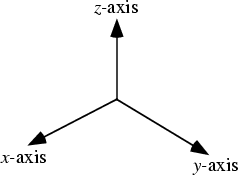Cartesian coordinates are rectilinear 2-D or 3-D coordinates (and therefore a special case of Curvilinear Coordinates) which are also called Rectangular Coordinates. The three axes of 3-D Cartesian coordinates, conventionally denoted the x-Axis-, y-Axis-, and z-Axis (a Notation due to Descartes) are chosen to be linear and mutually Perpendicular. In 3-D, the coordinates,, and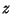may lie anywhere in the Interval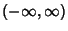.

The Scale Factors of Cartesian coordinates are all unity,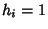. The Line Element is given by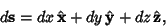(1)

and the Volume Element by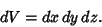(2)

The Gradient has a particularly simple form,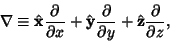(3)

as does the Laplacian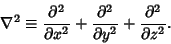(4)

The Laplacian is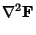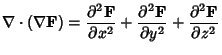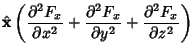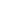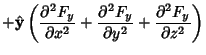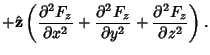(5)

The Divergence is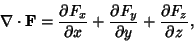(6)

and the Curl is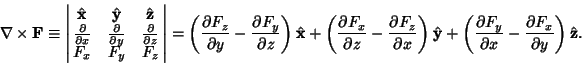(7)

The Gradient of the Divergence is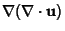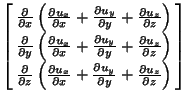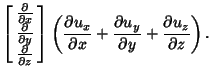(8)

Laplace's Equation is separable in Cartesian coordinates.

Arfken, G. Special Coordinate Systems--Rectangular Cartesian Coordinates.'' §2.3 in Mathematical Methods for Physicists, 3rd ed. Orlando, FL: Academic Press, pp. 94-95, 1985.